Courses

# Test: CAT Quant 2017 (Shift - 1)

## 34 Questions MCQ Test CAT New | Test: CAT Quant 2017 (Shift - 1)

Description
This mock test of Test: CAT Quant 2017 (Shift - 1) for CAT helps you for every CAT entrance exam. This contains 34 Multiple Choice Questions for CAT Test: CAT Quant 2017 (Shift - 1) (mcq) to study with solutions a complete question bank. The solved questions answers in this Test: CAT Quant 2017 (Shift - 1) quiz give you a good mix of easy questions and tough questions. CAT students definitely take this Test: CAT Quant 2017 (Shift - 1) exercise for a better result in the exam. You can find other Test: CAT Quant 2017 (Shift - 1) extra questions, long questions & short questions for CAT on EduRev as well by searching above.
QUESTION: 1

### Arun's present age in years is 40% of Barun's. In another few years, Arun's age will be half of Barun's. By what percentage will Barun's age increase during this period?

Solution:

Let Arun's current age be A. Hence, Barun's current age is 2.5A

Let Arun's age be half of Barun's age after X years.

Therefore, 2*(X+A) = 2.5A + X

or, X = 0.5A

Hence, Barun's age increased by 0.5A/2.5A = 20%

QUESTION: 2

### A person can complete a job in 120 days. He works alone on Day 1. On Day 2, he is joined by another person who also can complete the job in exactly 120 days. On Day 3, they are joined by another person of equal efficiency. Like this, everyday a new person with the same efficiency joins the work. How many days are required to complete the job?

Solution:

Let the rate of work of a person be x units/day. Hence, the total work = 120x.

It is given that one first day, one person works, on the second day two people work and so on.

Hence, the work done on day 1, day 2... will be x, 2x, 3x, ... respectively.

The sum should be equal to 120x.

120x = x * n(n+1)/2

n2 + n – 240 = 0

n = 15 is the only positive solution.

Hence, it takes 15 days to complete the work.

QUESTION: 3

### An elevator has a weight limit of 630 kg. It is carrying a group of people of whom the heaviest weighs 57 kg and the lightest weighs 53 kg. What is the maximum possible number of people in the group?

Solution:

It is given that the maximum weight limit is 630. The lightest person's weight is 53 Kg and the heaviest person's weight is 57 Kg.

In order to have maximum people in the lift, all the remaining people should be of the lightest weight possible, which is 53 Kg.

Let there be n people.

53 + n (53) + 57 = 630

n is approximately equal to 9.8. Hence, 9 people are possible.

Therefore, a total of 9 + 2 = 11 people can use the elevator.

QUESTION: 4

A man leaves his home and walks at a speed of 12 km per hour, reaching the railway station 10 minutes after the train had departed. If instead he had walked at a speed of 15 km per hour, he would have reached the station 10 minutes before the train's departure. The distance (in km) from his home to the railway station is

Solution:

We see that the man saves 20 minutes by changing his speed from 12 Km/hr to 15 Km/hr. Let d be the distance

Hence,

d/12 – d/15 = 1/3

d/60 = 1/3

d = 20 Km.

QUESTION: 5

Ravi invests 50% of his monthly savings in fixed deposits. Thirty percent of the rest of his savings is invested in stocks and the rest goes into Ravi's savings bank account. If the total amount deposited by him in the bank (for savings account and fixed deposits) is Rs 59500, then Ravi's total monthly savings (in Rs) is

Solution:

Let his total savings be 100x.

He invests 50x in fixed deposits. 30% of 50x, which is 15x is invested in stocks and 35x goes to savings bank.

It is given 85x = 59500

x = 700

Hence, 100x = 70000

QUESTION: 6

If a seller gives a discount of 15% on retail price, she still makes a profit of 2%. Which of the following ensures that she makes a profit of 20%?

Solution: Let the retail price be M and cost price be C.

Given,

0.85 M = 1.02 C

M = 1.2 C

If he wants 20% profit he has to sell at 1.2C, which is nothing but the retail price.

QUESTION: 7

A man travels by a motor boat down a river to his office and back. With the speed of the river unchanged, if he doubles the speed of his motor boat, then his total travel time gets reduced by 75%. The ratio of the original speed of the motor boat to the speed of the river is

Solution:

Let the speed of the river be and the speed of the boat be u. Let d be the one way distance and t be the initial time taken.

Given,

t = d/u-x + d/u+x ……. (i)

Also,

t/4 = d/2u-x + d/2u+x

t = 4d/2u-x + 4d/2u+x…… (ii)

Equating both i and ii,

d/u-x + d/u+x = 4d/2u-x + 4d/2u+x

2u/u2-x2 = 16u/(4u2 – x2)

4u2 – x2 = 8u2 – 8x2u2/x2 = 7/4

u/x = √6 /2

QUESTION: 8

Suppose, C1, C2, C3, C4, and C5 are five companies. The profits made by C1, C2, and C3 are in the ratio 9: 10: 8 while the profits made by C2, C4, and C5 are in the ratio 18 : 19 : 20. If C5 has made a profit of Rs 19 crore more than C1, then the total profit (in Rs) made by all five companies is

Solution: Given,

C1 : C2 : C3 = 9 : 10 : 8 ... i

C2 : C4 : C5 = 18 : 19 : 20 ... ii

Let's multiply i by 9 and ii by 5

C1 : C2 : C3 = 81 : 90 : 72

C2 : C4 : C5 = 90 : 95 : 100

Therefore, C1 : C2 : C3 : C4 : C5 = 81 : 90 : 72 : 95 : 100

Given,

100x - 81x = 19

x = 1

Hence, total profit = 100 + 95 + 72 + 90 + 81 = 438

QUESTION: 9

The number of girls appearing for an admission test is twice the number of boys. If 30% of the girls and 45% of the boys get admission, the percentage of candidates who do not get admission is

Solution: Let the number of girls be 2x and number of boys be x.

Number of students not getting admission = 3x - 0.6x -0.45x = 1.95x

Percentage = (1.95x/3x) * 100 = 65%

QUESTION: 10

A stall sells popcorn and chips in packets of three sizes: large, super, and jumbo. The numbers of large, super, and jumbo packets in its stock are in the ratio 7: 17: 16 for popcorn and 6: 15: 14 for chips. If the total number of popcorn packets in its stock is the same as that of chips packets, then the numbers of jumbo popcorn packets and jumbo chips packets are in the ratio

Solution: The ratio of L, S, J for popcorn = 7:17: 16

Let them be 7x, 17x and 16x

The ratio of L, S, J for chips = 6:15: 14

Let them 6y, 15y and 14y

Given, 40x = 35y, x= 7y/8

Jumbo popcor = 16x = 16 * 7y/8= 14y

Hence, the ratio of jumbo popcorn and jumbo chips = 1: 1

QUESTION: 11

In a market, the price of medium quality mangoes is half that of good mangoes. A shopkeeper buys 80 kg good mangoes and 40 kg medium quality mangoes from the market and then sells all these at a common price which is 10% less than the price at which he bought the good ones. His overall profit is

Solution: Let the cost of good mangoes be 2x per kg. The cost of medium mangoes be x per kg.

CP of good mangoes = 160x

CP of medium mangoes = 40x

His selling price = 0.9*2x = 1.80x

Therefore, total revenue generated by selling all the mangoes = 120*1.8x = 216x

Hence, the profit % =16x/200x * 100= 8%

QUESTION: 12

If Fatima sells 60 identical toys at a 40% discount on the printed price, then she makes 20% profit. Ten of these toys are destroyed in fire. While selling the rest, how much discount should be given on the printed price so that she can make the same amount of profit?

Solution: Let the cost price be C and the marked price be M.

Given,

0.6 M = 1.2 C

M = 2C

CP of 60 toys = 60C

Now only 50 are remaining.

Hence, M (1 - d) * 50 = 72C

1- d = 0.72

d = .28

Hence 28%

QUESTION: 13

If a and b are integers of opposite signs such that (a+3)2:b = 9:1 and (a-1)2 : (b-1)2 = 4:1, then the ratio a2 + b2 is

Solution: Since the square root can be positive or negative we will get two cases for each of the equation.

For the first one,

a + 3 = 3b .. i

a + 3 = -3b ... ii

For the second one,

a - 1 = 2(b -1) ... iii

a - 1 = 2 (1 - b) ... iv

we have to solve i and iii, i and iv, ii and iii, ii and iv.

Solving i and iii,

a + 3 = 3b and a = 2b - 1, solving, we get a = 3 and b = 2, which is not what we want.

Solving i and iv

a + 3 = 3b and a = 3 - 2b, solving, we get b = 1.2, which is not possible.

Solving ii and iii

a + 3 = -3b and a = 2b - 1, solving, we get b = 0.4, which is not possible.

Solving ii and iv,

a + 3 = -3b and a = 3 - 2b, solving, we get a = 15 and b = -6 which is what we want.

Thus, a2/b2 = 25/4

QUESTION: 14

A class consists of 20 boys and 30 girls. In the mid-semester examination, the average score of the girls was 5 higher than that of the boys. In the final exam, however, the average score of the girls dropped by 3 while the average score of the entire class increased by 2. The increase in the average score of the boys is

Solution: Let the average score of girls in the mid semester exam be G.

Therefore the average score of boys in the mid semester exam is G-5.

Hence, the total marks scored by the class is 30*G+20*(G-5) = 50*G – 100

Therefore, the average of the entire class is (50G-100)/50 = G-2

Therefore, the average of girls in the final exam is G-3 and the average of the entire class in the final exam is G.

Hence, the total marks scored by the class is 50G, while the total marks scored by the girls is 30*(G-3)=30G-90

Therefore, the total marks scored by the boys is 50G-(30G-90) = 20G+90

Hence, the average of the boys in the final exam is (20G+90)/20 = G+4.5

Hence, the increase in the average marks of the boys is (G+4.5)-(G-5) = 9.5

QUESTION: 15

The area of the closed region bounded by the equation I x I + I y I = 2 in the two-dimensional plane is

Solution: The following equation will form a square of side (2√2 )2

The area of the square = (2√2 )2 = 8 units.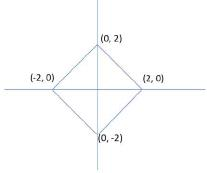QUESTION: 16

From a triangle ABC with sides of lengths 40 ft, 25 ft and 35 ft, a triangular portion GBC is cut off where G is the centroid of ABC. The area, in sq ft, of the remaining portion of triangle ABC is

Solution: The lengths are given as 40, 25 and 35.

The perimeter = 100

Semi-perimeter, s = 50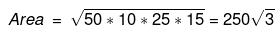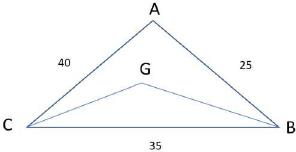The triangle formed by the centroid and two vertices is removed.

Since the cenroid divides the median in the ratio 2 : 1

The remaining area will be two-thirds the area of the original triangle.

Remaining area = 2 / 3 * 250√3 = 500 / √3.

QUESTION: 17

Let ABC be a right-angled isosceles triangle with hypotenuse BC. Let BQC be a semi-circle, away from A, with diameter BC. Let BPC be an arc of a circle centered at A and lying between BC and BQC.If AB has length 6 cm then the area, in sq cm, of the region enclosed by BPC and BQC is

Solution: The image of the figure is as shown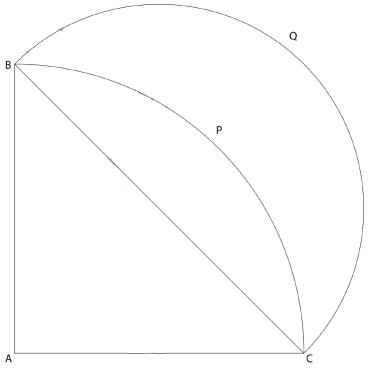AB = AC = 6cm. Thus, BC =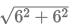= 6√2 cm

The required area = Area of semi-circle BQC - Area of quadrant BPC + Area of triangle ABC

Area of semicircle BQC

Diameter BC = 6√2cm

Radius = 6√2/2 = 3√2 cm

Area = πr2/2 = π*(3√2) /2 = 9π

Area = πr2/4 = π * (6)2 /4 = 9π

Area of triangle ABC

Area = 1/2 * 6 * 6 = 18

The required area = Area of semi-circle BQC - Area of quadrant BPC + Area of triangle ABC

= 9π - 9 π + 18 = 18

QUESTION: 18

A solid metallic cube is melted to form five solid cubes whose volumes are in the ratio 1: 1: 8: 27: 27. The percentage by which the sum of the surface areas of these five cubes exceeds the surface area of the original cube is nearest to

Solution: Let the volumes of the five cubes be x, x, 8x, 27x and 27x.

Let the sides be a, a, 2a, 3a and 3a. (x = a3)

Let the side of the original cube be A.

A3 = x+x+8x+27x+27x

A = 4a

Original surface area = 96a2

New surface area = 6(a2 + a2 + 4a2 + 9a2 + 9a2) = 144a2

Percentage increase = (144 – 96) / 96 * 100 = 50%

QUESTION: 19

A ball of diameter 4 cm is kept on top of a hollow cylinder standing vertically. The height of the cylinder is 3 cm, while its volume is cubic centimeters. Then the vertical distance, in cm, of the topmost point of the ball from the base of the cylinder is

Solution:

The volume of a cylinder is π *r2 *3 = 9π

r = √3 cm.

Radius of the ball is 2 cm. Hence, the ball will lie on top of the cylinder.

Lets draw the figure.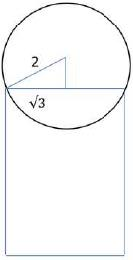Based on the Pythagoras theorem, the other leg will be 1 cm.

Thus, the height will be 3 + 1 + 2 = 6 cm

QUESTION: 20

Let ABC be a right-angled triangle with BC as the hypotenuse. Lengths of AB and AC are 15 km and 20 km, respectively. The minimum possible time, in minutes, required to reach the hypotenuse from A at a speed of 30 km per hour is

Solution:

The length of the altitude from A to the hypotenuse will be the shortest distance.

This is a right triangle with sides 3:4: 5.

Hence, the hypotenuse = = 25 Km.

Length of the altitude = = 12 Km

The time taken = 12/30 * 60 = 24 minutes

QUESTION: 21

Suppose, log3x = log12y = a, where x,y are positive numbers. If G is the geometric mean of x and y, and log6G is equal to

Solution:

We know that log3x = a and log12y = a

Hence, x=3a and y=12aTherefore, the geometric mean of and equals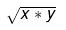This equals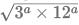= 6a

Hence, G = 6aOr, log6 G = a

QUESTION: 22

If x+1 = x2 and x>0, then 2x4 is

Solution:

We know that x2 – x – 1 =0

Therefore x4 = (x+1)2 = x2 + 2x + 1 = x + 1 + 2x + 1 = 3x + 2

Therefore, 2x4 = 6x + 4

We know that x>0 therefore, we can calculate the value of x to be 1+√5 /2

Hence, 2x4 = 6x + 4 = 3+3√5 +4 = 3√5 + 7

QUESTION: 23

The value of log0.008√5 + log√3 81 – 7 is equal to

Solution:

log0.008√5 + log√3 81 – 7

81 = 34 and 0.008

8/1000 = 23/103 = 1/53 = 5-3

Hence

log0.008√5+ 8 – 7

log5-351/2 + 8 – 7

log50.5/log5-3 + 1

1/-6 + 1

= 5/6

QUESTION: 24

If 92x-1 – 81x-1 = 1944, then x is

Solution:

81x/9 – 81x/81 = 1944

81x * [1/9 – 1/81] = 1944

81x * [8/81] = 243

34x = 39

x = 9/4

QUESTION: 25

The number of solutions (x,y,z) to the equation x – y – z = 25, where x, y, and z are positive integers such that x≤40,y≤12 and z≤12 is

Solution:

x - y - z = 25 and x≤40, y≤12, z≤12

If x = 40 then y + z = 15. Now since both y and z are natural numbers less than 12, so y can range from 3 to 12 giving us a total of 10 solutions. Similarly, if x = 39, then y + z = 14. Now y can range from 2 to 12 giving us a total of 11 solutions.

If x = 38, then y + z = 13. Now y can range from 1 to 12 giving us a total of 12 solutions.

If x = 37 then y + z = 12 which will give 11 solutions.

Similarly on proceeding in the same manner the number of solutions will be 10, 9, 8, 7 and so on till 1.

Hence, required number of solutions will be (1 + 2 + 3 + 4 . . . . + 12) + 10 + 11

= 12*13/2 + 21

78 + 21 = 99

QUESTION: 26

For how many integers n, will the inequality (n-5)(n-10)-3(n-2)≤0 be satisfied?

Solution:

(n-5) (n-10)-3(n-2) ≤ 2

=> n2 − 15n + 50 − 3n + 6 ≤ 0

=> n2 − 18n + 56 ≤ 0

=> (n − 4)(n − 14) ≤ 0

=> Thus, n can take values from 4 to 14. Hence, the required numbers of values are 14 - 4 + 1 = 11.

Positive Mark: 3

Negative Mark: 0

QUESTION: 27

If f1(x) = x2 + 11x + n and f2(x) = x, then the largest positive integer n for which the equation f1(x) = f2(x) has two distinct real roots is

Solution:

f1(x) = x2 + 11x + n and f2(x) = x,

f1(x) = f2(x)

x2 + 11x + n = x

x2 + 10x + n = 0

For this equation to have distinct real roots, b2 - 4ac>0

102> 4n

=> n < 100/4

=> n < 25

Thus, largest integral value that n can take is 24.

Positive Mark: 3

Negative Mark: 0

QUESTION: 28

If a,b,c and d are integers such that a+b+c+d then the minimum possible value of (a-b)2 + (a-c)2+(a-d)2 is

Solution:

For the value of given expression to be minimum, the values of and should be as close as possible. 30/4 = 7.5. Since each one of these are integers so values must be 8, 8, 7, 7. On putting these values in the given expression, we get

(8 − 8)2 + (8 − 7)2 + (8 − 7)2

=> 1 + 1 = 2

QUESTION: 29

Let AB, CD, EF, GH, and JK be five diameters of a circle with center at 0. In how many ways can three points be chosen out of A, B, C, D, E, F, G, H, J, K, and 0 so as to form a triangle?

Solution:

The total number of given points are 11. (10 on circumference and 1 is the center)

So total possible triangles = 11C3 = 165.

However, AOB, COD, EOF, GOH, JOK lie on a straight line. Hence, these 5 triangles are not possible. Thus, the required number of triangles = 165 - 5 = 160

QUESTION: 30

The shortest distance of the point (1/2, 1)from the curve y = I x -1I + I x + 1I is

Solution: The graph of the given function is as shown below.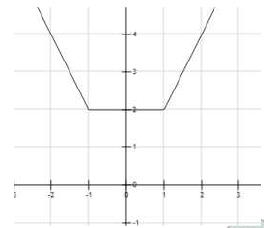We can see that the shortest distance of the point (1/2, 1) will be 1 unit.

QUESTION: 31

If the square of the 7th term of an arithmetic progression with positive common difference equals the product of the 3rd and 17th terms, then the ratio of the first term to the common difference is

Solution: The seventh term of an AP = a + 6d. Third term will be a + 2d and second term will be a + 16d. We are given that (a + 6d) 2 = (a + 2d) (a + 16d)

=>d: a = 3:2

we have been asked about a: d. Hence, it would be 2:3

QUESTION: 32

In how many ways can 7 identical erasers be distributed among 4 kids in such a way that each kid gets at least one eraser but nobody gets more than 3 erasers?

Solution: We have been given that a + b + c + d = 7

Total ways of distributing 7 things among 4 people so that each one gets at least one = n-1Cr-1 = 6C3 = 20 Now we need to subtract the cases where any one person got more than 3 erasers. Any person cannot get more than 4 erasers since each child has to get at least 1. Any of the 4 childs can get 4 erasers. Thus, there are 4 cases. On subtracting these cases from the total cases we get the required answer. Hence, the required value is 20 - 4 = 16

QUESTION: 33

f(x) = (5x + 2)/ (3x-5) and g(x) = x2 − 2x – 1, then the value of g(f(f(3))) is

Solution:

f (3) = (15 + 2) / (9 - 5) = 17/4

f (f (3)) = (5 * 17 / 4 + 2) / (3 * 17/ 4 – 5) = (93/4)/ (31/4) = 93/31 = 3

g(f (f (3))) = 32 − 3 ∗ 2 − 1 = 2

QUESTION: 34

Let a1, a2,.............,a3n be an arithmetic progression with a1 = 3 and a2 = 7. If a1 + a2 +...+a3n = 1830, then what is the smallest positive integer m such that m (a1 + a2 +...+an) > 1830?

Solution: a1= 3 and a2= 7. Hence, the common difference of the AP is 4.

We have been given that the sum up to 3n terms of this AP is 1830.

Hence, 1830 = m/2 [2*3 + (m – 1) *4]

=> 1830*2 = m(6 + 4m - 4)

=> 3660 = 2m + 4m2

=> 2m2 + m -1830 = 0

=> (m - 30)(2m + 61) = 0

=> m = 30 or m = -61/2

Since m is the number of terms so m cannot be negative. Hence, must be 30

So, 3n = 30

n = 10

Sum of the first '10' terms of the given AP = 5*(6 + 9*4) = 42*5 = 210

m(a1 +a2 +...+an) > 1830

=> 210m > 1830

=> m > 8.71

Hence, smallest integral value of 'm' is 9.